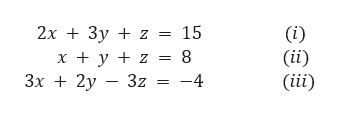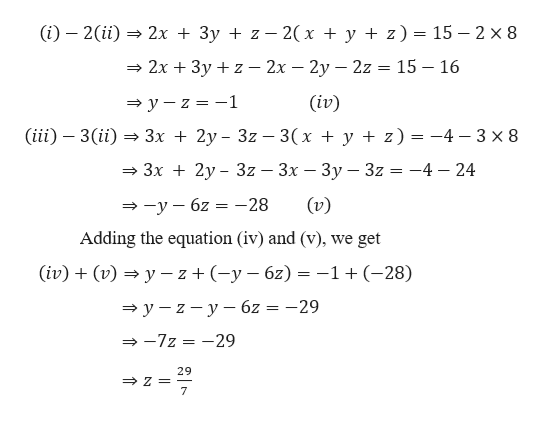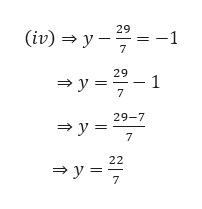# Solve the following system of equations:2x + 3y + z = 15x + y + z = 83x + 2y - 3z = -4

Question
3 views

Solve the following system of equations:
2x + 3y + z = 15
x + y + z = 8
3x + 2y - 3z = -4

check_circle

Step 1

Given,

The following system of equations:help_outlineImage Transcriptionclose2x 3y z 15 (i) (ii) (iii) = 8 x y z 3x2y 3z -4 fullscreen
Step 2

Solving the above system of equation by the elimination methodhelp_outlineImage Transcriptionclose(i) 2(ii2x + 3y z 2(x + y + z) = 15 2 x 8 2x 3y z - 2x - 2y - 2z = 15 - 16 (iv) y-z-1 (iii 3(ii 3x 2y-3z - 3(x + y + z) -4 3 x 8 = 3x 2y- 3z 3x - 3y - 3z = -4 - 24 (v) -y6z-28 Adding the equation (iv) and (v), we get (iv)(v)yz (-y6z-1+ (-28) y-z-y 6z = -29 -7z -29 29 Z 7 fullscreen
Step 3

Now, putting the value of z ...help_outlineImage Transcriptionclose29 (iv)y -1 7 29 1 y 7 29-7 y 7 22 y= 7 fullscreen

### Want to see the full answer?

See Solution

#### Want to see this answer and more?

Solutions are written by subject experts who are available 24/7. Questions are typically answered within 1 hour.*

See Solution
*Response times may vary by subject and question.
Tagged in

### Calculus